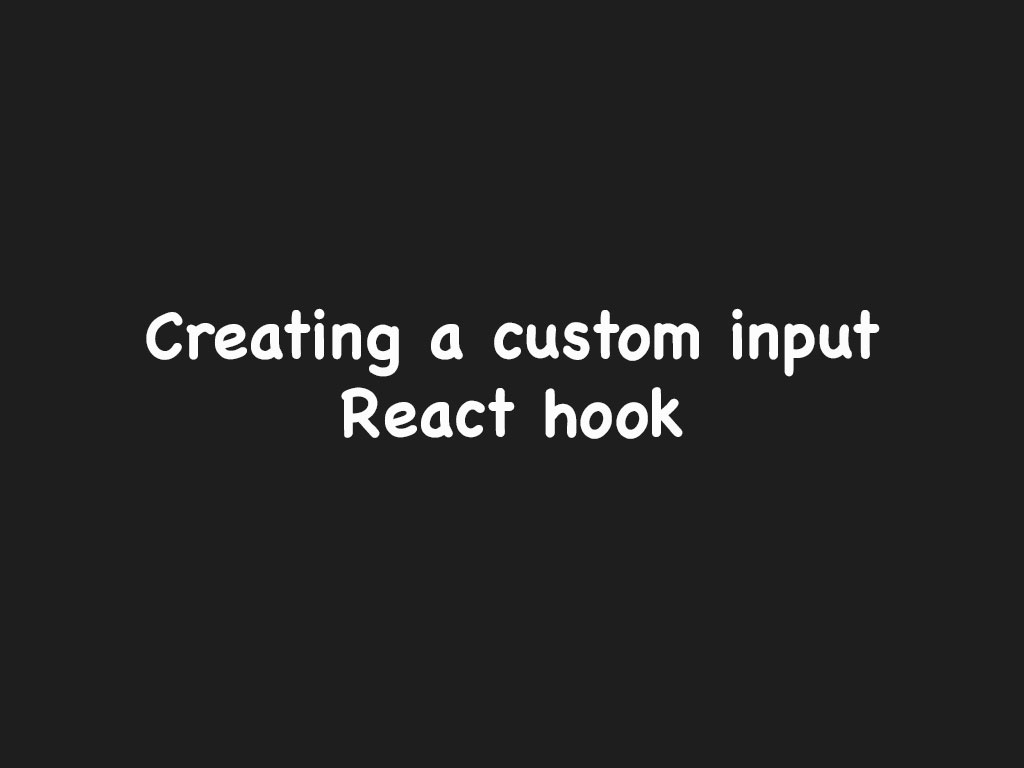# How to handle an input with React hooksWhen I first began to write form logic with React hooks, I would write my code as such:

``````
function App() {

return (
<>
<input type="text" placeholder="jdoe123" onChange={e => setUsername(e.target.value)} />
</>
);
}

``````

I realized that this was very repetitive code and got quickly annoyed. I was curious, is there a more efficient way to handle inputs with React hooks?

The answer is yes! We can write a reusable function that returns the input value of the `<input />` element itself.

Let’s get started in writing one!

## Solution: Writing an input with React hooks

The first step I’ll do is to create a function called `useInput`.

`useInput()` will accept an argument called `opts`, which will allow the developer to pass other input type of properties.

``````
function useInput(opts) {
const [value, setValue] = React.useState('');
const input = <input
value={value}
onChange={e => setValue(e.target.value)}
{...opts} />

return [value, input];
}
``````

Inside `useInput()` I will create a state hook that will contain the value of the input element and it will be updated `onChange event listener`. I will also create a variable called `input` that will hold the JSX element.

The return value will be tuple type, where the first element of the array is the value of the input element, and the second value will be the JSX element itself.

Now let’s see how it would be used in a parent component.

``````
function App() {

return (
<>